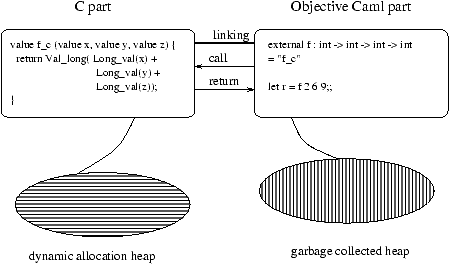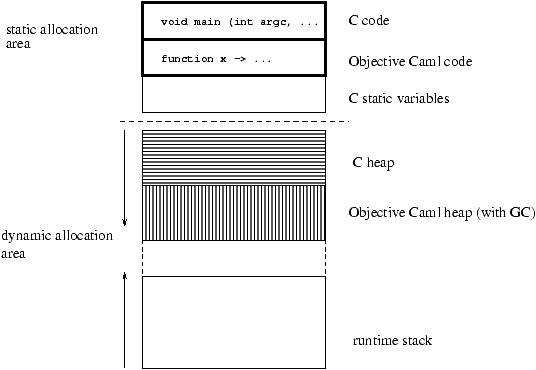## Communication between C and Objective CAML

Communication between parts of a program written in C and in Objective CAML is accomplished by creating an executable (or a new toplevel interpreter) containing both parts. These parts can be separately compiled. It is therefore the responsibility of the linking phase2 to establish the connection between Objective CAML function names and C function names, and to create the final executable. To this end, the Objective CAML part of the program contains external declarations describing this connection.

Figure 12.1 shows a sample program composed of a C part and an Objective CAML part.Figure 12.1: Communication between Objective CAML and C.

Each part comprises code (function definitions and toplevel expressions for Objective CAML) and a memory area for dynamic allocation. Calling the function f with three Objective CAML integer arguments triggers a call to the C function f_c. The body of the C function converts the three Objective CAML integers to C integers, computes their sum, and returns the result converted to an Objective CAML integer.

We now introduce the basic mechanisms for interfacing C with Objective CAML: external declarations, calling conventions for C functions invoked from Objective CAML, and linking options. Then, we show an example using input-output.

### External declarations

External function declarations in Objective CAML associate a C function definition with an Objective CAML name, while giving the type of the latter.

The syntax is as follows:

### Syntax

external caml_name : type = "C_name"
This declaration indicates that calling the function caml_name from Objective CAML code performs a call to the C function C_name with the given arguments. Thus, the example in figure 12.1 declares the function f as the Objective CAML equivalent of the C function f_c.

An external function can be declared in an interface (i.e., in an .mli file) either as an external or as a regular value:

### Syntax

 external caml_name : type = "C_name" val caml_name : type

In the latter case, calls to the C function first go through the general function application mechanism of Objective CAML. This is slightly less efficient, but hides the implementation of the function as a C function.

### Declaration of the C functions

C functions intended to be called from Objective CAML must have the same number of arguments as described in their external declarations. These arguments have type value, which is the C type for Objective CAML values. Since those values have uniform representations (9), a single C type suffices to encode all Objective CAML values. On page ??, we will present the facilities for encoding and decoding values, and illustrate them by a function that explores the representations of Objective CAML values.

The example in figure 12.1 respects the constraints mentioned above. The function f_c, associated with an Objective CAML function of type int -> int -> int -> int, is indeed a function with three parameters of type value returning a result of type value.

The Objective CAML bytecode interpreter evaluates calls to external functions differently, depending on the number of arguments3. If the number of arguments is less than or equal to five, the arguments are passed directly to the C function. If the number of arguments is greater than five, the C function's first parameter will get an array containing all of the arguments, and the C function's second parameter will get the number of arguments. These two cases must therefore be distinguished for external C functions that can be called from the bytecode interpreter. On the other hand, the Objective CAML native-code compiler always calls external functions by passing all the arguments directly, as function parameters.

#### External functions with more than five arguments

For external functions with more than five arguments, the programmer must provide two C functions: one for bytecode and the other for native-code. The syntax of external declarations allows the declaration of one Objective CAML function associated with two C functions:

### Syntax

external caml_name : type = "C_name_bytecode" "C_name_native"
The function C_name_bytecode takes two parameters: an array of values of type value (i.e. a C pointer of type value*) and an integer giving the number of elements in this array.

#### Example

The following C program defines two functions for adding together six integers: plus_native, callable from native code, and plus_bytecode, callable from the bytecode compiler. The C code must include the file mlvalues.h containing the definitions of C types, Objective CAML values, and conversion macros.

````#include <stdio.h>``#include <caml/mlvalues.h>``value plus_native (value x1,value x2,value x3,value x4,value x5,value x6)``{``  printf("<< NATIVE PLUS >>\n") ; fflush(stdout) ;``  return Val_long ( Long_val(x1) + Long_val(x2) + Long_val(x3)``                  + Long_val(x4) + Long_val(x5) + Long_val(x6)) ;``}``value plus_bytecode (value * tab_val, int num_val)``{``  int i;``  long res;``  printf("<< BYTECODED PLUS >> : ") ; fflush(stdout) ;``  for (i=0,res=0;i<num_val;i++) res += Long_val(tab_val[i]) ;``  return Val_long(res) ;``}`

```
The following Objective CAML program exOCAML.ml calls these two C functions.
```external` `plus` ``:`` `int` `->` `int` `->` `int` `->` `int` `->` `int` `->` `int` `->` `int` `` `` `` `` `` `` `` `` `` `` `` `` `` `` ``=`` ``"plus_bytecode"`` ``"plus_native"`` `;;print_int` `(plus` ``1`` ``2`` ``3`` ``4`` ``5`` ``6`)` `;;print_newline` `()` `;;

```

We now compile these programs with the two Objective CAML compilers and a C compiler that we call cc. We must give it the access path for the mlvalues.h include file.
```\$ cc -c -I/usr/local/lib/ocaml  exC.c

\$ ocamlc -custom exC.o exOCAML.ml -o ex_byte_code.exe
\$ ex_byte_code.exe
<< BYTECODED PLUS >> : 21

\$ ocamlopt exC.o exOCAML.ml -o ex_native.exe
\$ ex_native.exe
<< NATIVE PLUS >> : 21
```

### Note

To avoid writing the C function twice (with the same body but different calling conventions), it suffices to implement the bytecode version as a call to the native-code version, as in the following sketch:
value prim_nat (value x1, ..., value xn) { ... }
value prim_bc (value *tbl, int n)
{ return prim_nat(tbl,tbl,...,tbl[n-1]) ; }

The linking phase creates an executable from C and Objective CAML files compiled with their respective compilers. The result of the native-code compiler is shown in figure 12.2.Figure 12.2: Mixed-language executable.

The compilation of the C and Objective CAML sources generates machine code that is stored in the static allocation area of the program. The dynamic allocation area contains the execution stack (corresponding to the function calls in progress) and the heaps for C and Objective CAML.

#### Run-time libraries

The C functions that can be called from a program using only the standard Objective CAML library are contained in the execution library of the abstract machine (see figure 7.3 page ??). For such a program, there is no need to provide additional libraries at link-time. However, when using Objective CAML libraries such as Graphics, Num or Str, the programmer must explicitly provide the corresponding C libraries at link-time. This is the purpose of the -custom compiler option (see 7, page ??). Similarly, when we wish to call our C functions from Objective CAML, we must provide the object file containing those C functions at link-time. The following example illustrates this.

The linking commands differ slightly between the native-code compiler, the bytecode compiler, and the construction of toplevel interactive loops. The compiler options relevant to these linking modes are described in chapter 7.

To illustrate these linking modes, we consider again the example in figure 12.1. Assume the Objective CAML source file is named progocaml.ml. It uses the external function f_c defined in the C file progC.c. In turn, the function f_c refers to a C library a_C_library.a. Once all these files are compiled separately, we link them together using the following commands:
• bytecode:
`ocamlc -custom -o vbc.exe progC.o a_C_library.a progocaml.cmo`
• native code:
`ocamlopt progC.o -o vn.exe a_C_library.a progocaml.cmx`
We obtain two executable files: vbc.exe for the bytecode version, and vn.exe for the native-code version.

#### Building an enriched abstract machine

Another possibility is to augment the run-time library of the abstract machine with new C functions callable from Objective CAML. This is achieved by the following commands:
```ocamlc -make-runtime -o new_ocamlrun progC.o a_C_library.a
```
We can then build a bytecode executable vbcnam.exe targeted to the new abstract machine:
```ocamlc -o vbcnam.exe -use-runtime new_ocamlrun progocaml.cmo
```
To run this bytecode executable, either give it as the first argument to the new abstract machine, as in `new_ocaml vbcnam.exe` , or run it directly as `vbcnam.exe`

### Note

Linking in -custom mode scans the object files (.cmo) to build a table of all external functions mentioned. The bytecode required to use them is generated and added to the bytecode corresponding to the Objective CAML code.

#### Building a toplevel interactive loop

To be able to use an external function in the toplevel interactive loop, we must first build a new toplevel interpreter containing the C code for the function, as well as an Objective CAML file containing its declaration.

We assume that we have compiled the file progC.c containing the function f_c. We then build the toplevel loop ftop as follows:
```ocamlmktop -custom -o ftop progC.o a_C_library.a ex.ml
```
The file ex.ml contains the external declaration for the function f. The new toplevel interpreter ftop then knows this function and contains the corresponding C code, as found in progC.o.

### Mixing input-output in C and in Objective CAML

The input-output functions in C and in Objective CAML do not share their file buffers. Consider the following C program:

````#include <stdio.h>``#include <caml/mlvalues.h>``value hello_world (value v)``  { printf("Hello World !!");  fflush(stdout);  return v; }`

```
Writes to standard output must be flushed explicitly (fflush) to guarantee that they will be printed in the intended order.

```# external` `caml_hello_world` ``:`` `unit` `->` `unit` ``=`` ``"hello_world"`` `` `;;`external caml_hello_world : unit -> unit = "hello_world"`# print_string` ``"<< "`` `;` `caml_hello_world` `()` `;` `print_string` ``" >>\n"`` `;` `flush` `stdout` `;;`Hello World !!<<  >>``- : unit = ()`

```

The outputs from C and from Objective CAML are not intermingled as expected, because each language buffers its outputs independently. To get the correct behavior, the Objective CAML part must be rewritten as follows:
```# print_string` ``"<< "`` `;` `flush` `stdout` `;` `caml_hello_world` `()` `;` `` `print_string` ``" >>\n"`` `;` `flush` `stdout` `;;`<< Hello World !! >>``- : unit = ()`

```
By flushing the Objective CAML output buffer after each write, we ensure that the outputs from each language appear in the expected order.